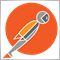Array count elements of the same value and add to object - page 22391

I was bored while waiting for a trade so here's a class that takes an array of doubles and makes a descending sorted list by number of occurrences using the optimized algorithm above.

#include <MyDataStructures\OccurrenceList.mqh>
void OnStart()
{
double pivots[];
int total = ArrayResize(pivots,Bars);
for(int i=0;i<total;i++)
pivots[i] = (High[i]+Low[i]+Close[i])/3.0;

COccurrenceList list;
list.ArrayIn(pivots,  //pivot point array
100,    //round to 100 points
5      //minimum count threshold = 5 occurrences
);

for(int i=0;i<10&&i<list.Total();i++)
printf("The number of times %s occurred as Pivot = %d",
DoubleToString(list[i].value,_Digits),list[i].count);
}
0       13:46:16.792    Foo USDJPY_,H1: The number of times 113.500 occurred as Pivot = 315
0       13:46:16.792    Foo USDJPY_,H1: The number of times 112.500 occurred as Pivot = 299
0       13:46:16.792    Foo USDJPY_,H1: The number of times 111.500 occurred as Pivot = 254
0       13:46:16.792    Foo USDJPY_,H1: The number of times 112.700 occurred as Pivot = 222
0       13:46:16.792    Foo USDJPY_,H1: The number of times 114.000 occurred as Pivot = 210
0       13:46:16.792    Foo USDJPY_,H1: The number of times 112.800 occurred as Pivot = 205
0       13:46:16.792    Foo USDJPY_,H1: The number of times 112.000 occurred as Pivot = 204
0       13:46:16.792    Foo USDJPY_,H1: The number of times 113.400 occurred as Pivot = 200
0       13:46:16.792    Foo USDJPY_,H1: The number of times 111.400 occurred as Pivot = 198
0       13:46:16.792    Foo USDJPY_,H1: The number of times 113.000 occurred as Pivot = 197

Files: